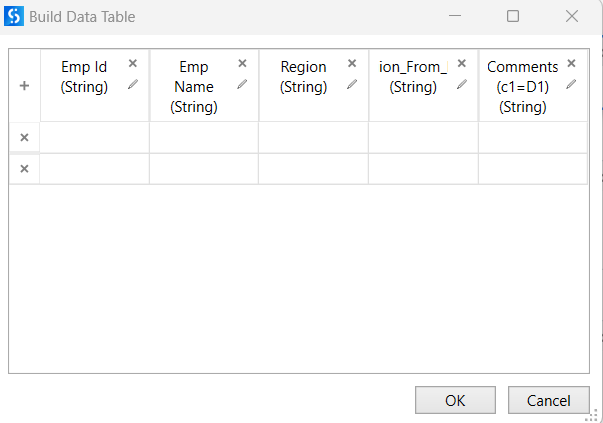# How to compare between two excels without forloop

Hi Team ,
What is the simple way to add few columns from one excel sheet & few from other & add new columns where some calculations needs to be made based on these two copied columns.

Example : emp Id , emp name , region from one excel sheet & copy region from other excel & add a new column ‘Remarks/comments’ which will hold True/False based on comparisons between those two regions.

If you can elaborate a little bit more, on the region column how does it add? Is there any key based that will match, for example, emp ID? or else it will simply add the region column into the table?

Solve it in excel itself.
Create the column containing a cell formula with an if statement.
=if(\$A1=\$B1, TRUE, FALSE)
(make the row numbers dynamic ofc.).

You can set this formula through UiPath but that would probably still involve some loop.
Or predefine an excel template already containing that formula (or an extended version where needed) and just output your data into that template.

Alternatively you could also use vlookups, then you don’t even have to transcribe the data between the different sheets if done well.

Example my first table dt1 has

Emp ID Emp Name Region
123 abc Ind
456 xyz US

and second table has

Emp Code Region
123 Ind
345 UK

and I need Output like this

Emp ID Emp Name Region Region from dt2 Comments(c1=D1)
123 abc Ind Ind True
456 xyz US NA False

Yeah but first I need to copy first two columns from data table next two from another & then I can apply the cell formula which you told.
So as per my below sample data tables can you suggest how can we do without loop using Linq/lamda

Can you check is it working for you?

build datatable- dtOutputassign
dtOutput = (From a In dtEmp_Details Join b In dtRegion On a(“Emp Id”).toString Equals b(“Emp Code”).ToString Select dtOutput.Rows.Add({a(“Emp Id”),a(“Emp Name”),a(“Region”),b(“Region”)})).CopyToDatatable

assign

Thanks

@Divya_Salve
parameters for dt3 in/out direction
by using invoke code activity we can…

For Each row1 As datarow In dt1.AsEnumerable
For Each row2 As DataRow In dt2.AsEnumerable
If(row1(2).ToString=(row2(1).ToString)) Then
Exit For
Else
Exit For
End If
Next
Next

build a dt3 with required feilds

@Divya_Salve

1. Join the datatable dt1 and dt2 on left join
3. To fill the true or false…use the below code in invoke code with passing dt as in/out variable where this is the joined datatable

`dt.AsEnumerable.ToList.ForEach(Sub(r) r("New Column") = If(String.IsNullOrEmpty(r("EmpCode").ToString),"False","True"))`

1. Filter datatable to remove the columns you dont need

Cheers

Hey , thanks for Quick Reply but this logic only compares the regions right?
But we have compare on both Emp ID & Region , now with this logic it will show true even thought the emp Id’s are diff in dt1 & dt2.

Try These Steps:

1.Use Read Range Acitvity to get data from first excel sheet ,save output in dt1
2. Use Read Range Acitvity to get data from second excel sheet ,save output in dt2
3.Use Build DataTable Activity to create final DataTable format with required columns eg: emp Id , emp name , region1, region2, comments
3. Use Linq to Copy required cells from dt1 and dt2 to Result table

(From row1 In dt1.AsEnumerable() Join row2 In dt2.AsEnumerable() On row1(“emp Id”) Equals row2(“emp Id”) Select Result.Rows.Add(row1(“emp Id”), row1(“emp name”), row1(“region1”),row2(“region2”))).CopyToDataTable

1. Assign True and False in Comment Column using linkq

(From row In resultTable.AsEnumerable() Select resultTable.Clone().LoadDataRow({row(“region1”), row(“region2”), If(row(“region1”).ToString = row(“region2”).ToString, “True”, “False”)}, False)).CopyToDataTable()